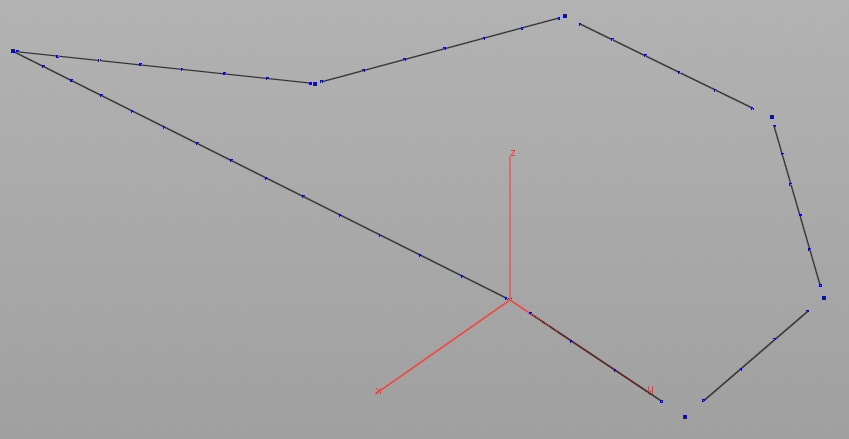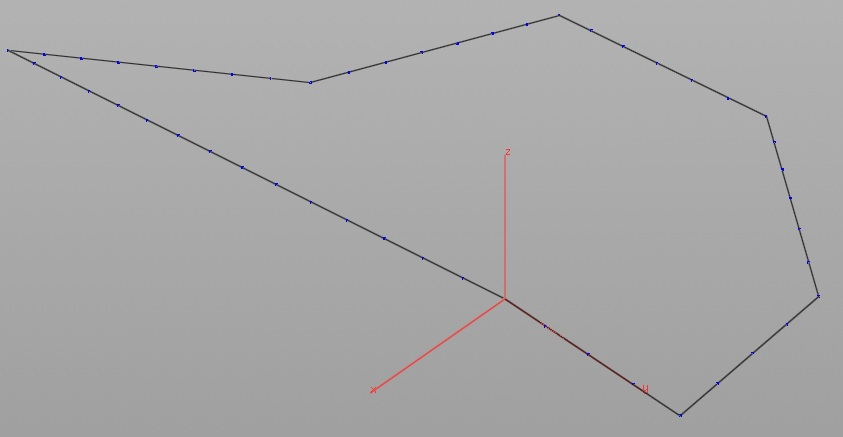# How to connect points to other points by attributes?

## Recommended Posts

Good day everyone!
For now I can introduce you another, I guess, simple thing.
it's a fully procedural model and I'm trying to connect all "corner points" to all "wall primitives" and get one close shape with same amount and position of points on this shape.
Below attached pictures of what I have at the current time and how (as example) that expecting to be at the end.
Does anybody know how to solve that stage? Any possibility to do that with SOP\VOP nodes, or VEX is essential?
Thank you##### Share on other sites

Hi,

one possible solution can be connecting the points by neighbourcount (attribute). To avoid connecting the wrong points, all possible distances between all point pairs can be sorted to define a maximum distance from the right value of the sorted array.

##### Share on other sites
33 minutes ago, Aizatulin said:

Hi,

one possible solution can be connecting the points by neighbourcount (attribute). To avoid connecting the wrong points, all possible distances between all point pairs can be sorted to define a maximum distance from the right value of the sorted array.

With this code in "attribWrangler" node I have that kind of issues:

int n = npoints(0);
int zero[];
int one[];
int c;
// zero ~ isolated points
// one ~ end points (with only one neighbour)
for (int i = 0; i < n; i++)
{
c = point(0, 'n', i);
if (c == 0)
{
push(zero, i);
}
if (c == 1)
{
push(one, i);
}
}

n = len(zero);
int l = len(one);
float dist[];
vector V,W;
float d;
// calculate all possible distances between zero and one
for (int i = 0; i < n; i++)
{
V = point(0, 'P', zero);
for (int k = 0; k < l; k++)
{
W = point(0, 'P', one[k]);
d = length(W - V);
push(dist, d);
}
}
// sort distances
sort(dist);
// the n * 2 - smallest value should be the maximum distance
float maxdist = dist[n * 2 - 1];

int line;
// now connect all points with a smaller distance than maxdist
// zero <-> one[k], if distance is small enough
for (int i = 0; i < n; i++)
{
V = point(0, 'P', zero);
for (int k = 0; k < l; k++)
{
W = point(0, 'P', one[k]);
d = length(W - V);
if (d <= maxdist)
{
}
}
}
____________________________________________

Issues:
Error:       The sub-network output operator failed to cook: /obj/curve_object1/by_dist/attribvop1
Error:       Vex error: /obj/curve_object1/by_dist/attribvop1/snippet1: Syntax error, unexpected end of file. (46,27)
Failed to resolve VEX code op:/obj/curve_object1/by_dist/attribvop1.

...On top of that I could say that I am absolutely zero in scripting... :c
Maybe that errors cause I use old version of Houdini (v13) cause of my PC.##### Share on other sites

one way of doing this would be,

with the a couple of foreach loop nodes every line would have a start which is 0 and the end, number of points -1 , and then you can use the fuse sop to connect between the points , this is the bear bones of achieving this

or

search closest point and add a line , fuse them , resample points, file below

joinconnect.hiplc

Edited by pxptpw

##### Share on other sites
On 11/23/2018 at 2:40 PM, pxptpw said:

one way of doing this would be,

with the a couple of foreach loop nodes every line would have a start which is 0 and the end, number of points -1 , and then you can use the fuse sop to connect between the points , this is the bear bones of achieving this

or

search closest point and add a line , fuse them , resample points, file below

...My H v13 cannot open this file, sorry >.<

##### Share on other sites

you could use the Polypath node. Might have to use a join node first after merging.

•1

## Create an account

Register a new account